# A multiple Hilbert-type integral inequality with a non-homogeneous kernel

## Abstract

By using the way of weight functions and the technic of real analysis, a multiple Hilbert-type integral inequality with a non-homogeneous kernel is given. The operator expression with the norm, the reverses and some examples with the particular kernels are also considered.

MSC:47A07, 26D15.

## 1 Introduction

If $p>1$, $\frac{1}{p}+\frac{1}{q}=1$, $f\left(\ge 0\right)\in {L}^{p}\left(0,\mathrm{\infty }\right)$, $g\left(\ge 0\right)\in {L}^{q}\left(0,\mathrm{\infty }\right)$, ${\parallel f\parallel }_{p},{\parallel g\parallel }_{q}>0$, then we have the following equivalent inequalities (cf. ):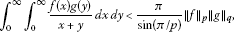(1)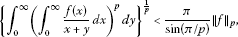(2)

where the constant factor $\frac{\pi }{sin\left(\pi /p\right)}$ is the best possible. (1) is the well-known Hardy-Hilbert integral inequality. Define the Hardy-Hilbert integral operator $T:{L}^{p}\left(0,\mathrm{\infty }\right)\to {L}^{p}\left(0,\mathrm{\infty }\right)$ as follows: for $f\in {L}^{p}\left(0,\mathrm{\infty }\right)$, $Tf\left(y\right):={\int }_{0}^{\mathrm{\infty }}\frac{1}{x+y}f\left(x\right)\phantom{\rule{0.2em}{0ex}}dx$ ($y\in \left(0,\mathrm{\infty }\right)$).

Then in view of (2), it follows ${\parallel Tf\parallel }_{p}<\frac{\pi }{sin\left(\pi /p\right)}{\parallel f\parallel }_{p}$ and $\parallel T\parallel \le \frac{\pi }{sin\left(\pi /p\right)}$. Since the constant is the best possible, we find $\parallel T\parallel =\frac{\pi }{sin\left(\pi /p\right)}$.

Inequalities (1) and (2) and the operator are important in analysis and its applications (cf. [2, 3]). In 2002,  considered the property of the Hardy-Hilbert integral operator and gave an improvement of (1) (for $p=q=2$). In 2004, by introducing another pair of conjugate exponents $\left(r,s\right)$ ($r>1$, $\frac{1}{r}+\frac{1}{s}=1$) and an independent parameter $\lambda >0$,  gave the best extensions of (1) as follows:

${\int }_{0}^{\mathrm{\infty }}{\int }_{0}^{\mathrm{\infty }}\frac{f\left(x\right)g\left(y\right)}{{x}^{\lambda }+{y}^{\lambda }}\phantom{\rule{0.2em}{0ex}}dx\phantom{\rule{0.2em}{0ex}}dy<\frac{\pi }{\lambda sin\left(\pi /r\right)}{\parallel f\parallel }_{p,\varphi }{\parallel g\parallel }_{q,\psi },$
(3)

where $\varphi \left(x\right)={x}^{p\left(1-\frac{\lambda }{r}\right)-1}$, $\psi \left(x\right)={x}^{q\left(1-\frac{\lambda }{s}\right)-1}$, ${\parallel f\parallel }_{p,\varphi }={\left\{{\int }_{0}^{\mathrm{\infty }}\varphi \left(x\right){f}^{p}\left(x\right)\phantom{\rule{0.2em}{0ex}}dx\right\}}^{\frac{1}{p}}>0$, ${\parallel g\parallel }_{q,\psi }>0$. In 2007,  gave the following inequality with the best constant $B\left(\frac{\lambda }{2},\frac{\lambda }{2}\right)$ ($\lambda >0$; $B\left(u,v\right)$ is the beta function):

${\int }_{0}^{\mathrm{\infty }}{\int }_{0}^{\mathrm{\infty }}\frac{f\left(x\right)g\left(y\right)}{{\left(1+xy\right)}^{\lambda }}\phantom{\rule{0.2em}{0ex}}dx\phantom{\rule{0.2em}{0ex}}dy
(4)

In 2009,  gave an extension of (4) in ${\mathbf{R}}^{2}$ with the kernel $\frac{1}{{|1+xy|}^{\lambda }}$ ($0<\lambda <1$);  gave another extension of (4) to the general kernel ${k}_{\lambda }\left(1,xy\right)$ ($\lambda >0$) with a pair of conjugate exponents $\left(p,q\right)$ and obtained the following multiple Hilbert-type integral inequality. Suppose that $n\in \mathbf{N}\mathrm{\setminus }\left\{1\right\}$, ${p}_{i}>1$, ${\sum }_{i=1}^{n}\frac{1}{{p}_{i}}=1$, $\lambda >0$, ${k}_{\lambda }\left({x}_{1},\dots ,{x}_{n}\right)\ge 0$ is a measurable function of −λ-degree in ${\mathbf{R}}_{+}^{n}$, and for any $\left({r}_{1},\dots ,{r}_{n}\right)$ (${r}_{i}>1$), satisfies ${\sum }_{i=1}^{n}\frac{1}{{r}_{i}}=1$ and

${k}_{\lambda }={\int }_{{\mathbf{R}}_{+}^{n-1}}{k}_{\lambda }\left({u}_{1},\dots ,{u}_{n-1},1\right)\prod _{j=1}^{n-1}{u}_{j}^{\frac{\lambda }{{r}_{j}}-1}\phantom{\rule{0.2em}{0ex}}d{u}_{1}\cdots d{u}_{n-1}>0.$

If ${\varphi }_{i}\left(x\right)={x}^{{p}_{i}\left(1-\frac{\lambda }{{r}_{i}}\right)-1}$, ${f}_{i}\left(\ge 0\right)\in {L}_{{\varphi }_{i}}^{{p}_{i}}\left(0,\mathrm{\infty }\right)$, ${\parallel f\parallel }_{{p}_{i,{\varphi }_{i}}}>0$ ($i=1,\dots ,n$), then we have the following inequality:

${\int }_{{\mathbf{R}}_{+}^{n}}{k}_{\lambda }\left({x}_{1},\dots ,{x}_{n}\right)\prod _{i=1}^{n}{f}_{i}\left({x}_{i}\right)\phantom{\rule{0.2em}{0ex}}d{x}_{1}\cdots d{x}_{n}<{k}_{\lambda }\prod _{i=1}^{n}{\parallel {f}_{i}\parallel }_{{p}_{i},{\varphi }_{i}},$
(5)

where the constant factor ${k}_{\lambda }$ is the best possible. For $n=2$, ${k}_{\lambda }\left(x,y\right)=\frac{1}{{x}^{\lambda }+{y}^{\lambda }}$ in (5), we obtain (3). Inequality (5) is an extension of the results in  and . In recent years,  and  considered some Hilbert-type operators relating (1)-(3);  also considered a multiple Hilbert-type integral operator with the homogeneous kernel of $-n+1$-degree and the relating particular case of (5) (for $\lambda =n-1$, $\frac{1}{{r}_{i}}=\frac{1}{n-1}\left(1-\frac{1}{{p}_{i}}\right)$).

In this paper, by using the way of weight functions and the technic of real analysis, a multiple Hilbert-type integral inequality with a non-homogeneous kernel is given. The operator expression with the norm, the reverses and some examples with the particular kernels are considered.

## 2 Some lemmas

Lemma 1 If $n\in \mathbf{N}\mathrm{\setminus }\left\{1\right\}$, ${\lambda }_{i}\in \mathbf{R}$ ($i=1,\dots ,n$), ${\sum }_{i=1}^{n}\frac{1}{{p}_{i}}=1$, then we have

$A:=\prod _{i=1}^{n}{\left[{x}_{i}^{\left({\lambda }_{i}-1\right)\left(1-{p}_{i}\right)}\prod _{j=1\left(j\ne i\right)}^{n}{x}_{j}^{{\lambda }_{j}-1}\right]}^{\frac{1}{{p}_{i}}}=1.$
(6)

Proof

We find

$\begin{array}{rcl}A& :=& \prod _{i=1}^{n}{\left[{x}_{i}^{\left({\lambda }_{i}-1\right)\left(1-{p}_{i}\right)+1-{\lambda }_{i}}\prod _{j=1}^{n}{x}_{j}^{{\lambda }_{j}-1}\right]}^{\frac{1}{{p}_{i}}}\\ =& \prod _{i=1}^{n}{\left[{x}_{i}^{\left(1-{\lambda }_{i}\right){p}_{i}}\prod _{j=1}^{n}{x}_{j}^{{\lambda }_{j}-1}\right]}^{\frac{1}{{p}_{i}}}=\prod _{i=1}^{n}{x}_{i}^{1-{\lambda }_{i}}{\left(\prod _{j=1}^{n}{x}_{j}^{{\lambda }_{j}-1}\right)}^{{\sum }_{i=1}^{n}\frac{1}{{p}_{i}}},\end{array}$

and then (6) is valid. □

Definition 1 If $n\in \mathbf{N}$, ${\mathbf{R}}_{+}^{n}:=\left\{\left({x}_{1},\dots ,{x}_{n}\right)|{x}_{i}>0\phantom{\rule{0.25em}{0ex}}\left(i=1,\dots ,n\right)\right\}$, $\lambda \in \mathbf{R}$, ${k}_{\lambda }\left({x}_{1},\dots ,{x}_{n}\right)$ is a measurable function in ${\mathbf{R}}_{+}^{n}$ such that for any $u>0$ and $\left({x}_{1},\dots ,{x}_{n}\right)\in {\mathbf{R}}_{+}^{n}$, ${k}_{\lambda }\left(u{x}_{1},\dots ,u{x}_{n}\right)={u}^{-\lambda }{k}_{\lambda }\left({x}_{1},\dots ,{x}_{n}\right)$, then we call ${k}_{\lambda }\left({x}_{1},\dots ,{x}_{n}\right)$ the homogeneous function of −λ-degree in ${\mathbf{R}}_{+}^{n}$.

Lemma 2 Suppose $n\in \mathbf{N}\mathrm{\setminus }\left\{1\right\}$, ${\lambda }_{i}\in \mathbf{R}$ ($i=1,\dots ,n$), ${\lambda }_{n}={\sum }_{i=1}^{n-1}{\lambda }_{i}=\frac{\lambda }{2}$, ${k}_{\lambda }\left({x}_{1},\dots ,{x}_{n}\right)\ge 0$ is a homogeneous function ofλ-degree. If

$\begin{array}{rcl}H\left(i\right)& :=& {\int }_{{\mathbf{R}}_{+}^{n-1}}{k}_{\lambda }\left({u}_{1},\dots ,{u}_{i-1},1,{u}_{i+1},\dots ,{u}_{n}\right)\\ ×\prod _{j=1\left(j\ne i\right)}^{n}{u}_{j}^{{\lambda }_{j}-1}\phantom{\rule{0.2em}{0ex}}d{u}_{1}\cdots d{u}_{i-1}\phantom{\rule{0.2em}{0ex}}d{u}_{i+1}\cdots d{u}_{n}\phantom{\rule{1em}{0ex}}\left(i=1,\dots ,n\right)\end{array}$

satisfying ${k}_{\lambda }:=H\left(n\right)\in \mathbf{R}$, then each $H\left(i\right)=H\left(n\right)={k}_{\lambda }$ and for any $i=1,\dots ,n$,

$\begin{array}{rcl}{\omega }_{i}\left({x}_{i}\right)& :=& {x}_{i}^{{\lambda }_{i}}{\int }_{{\mathbf{R}}_{+}^{n-1}}{k}_{\lambda }\left({x}_{1}{x}_{n},\dots ,{x}_{n-1}{x}_{n},1\right)\\ ×\prod _{j=1\left(j\ne i\right)}^{n}{x}_{j}^{{\lambda }_{j}-1}\phantom{\rule{0.2em}{0ex}}d{x}_{1}\cdots d{x}_{i-1}\phantom{\rule{0.2em}{0ex}}d{x}_{i+1}\cdots d{x}_{n}={k}_{\lambda }\phantom{\rule{1em}{0ex}}\left(\in \mathbf{R}\right).\end{array}$
(7)

Proof Setting ${u}_{j}={u}_{n}{v}_{j}$ ($j\ne i,n$) in the integral $H\left(i\right)$, we find

$\begin{array}{rcl}H\left(i\right)& =& {\int }_{{\mathbf{R}}_{+}^{n-1}}{k}_{\lambda }\left({v}_{1},\dots ,{v}_{i-1},{u}_{n}^{-1},{v}_{i+1},\dots ,{v}_{n-1},1\right)\prod _{j=1\left(j\ne i\right)}^{n-1}{v}_{j}^{{\lambda }_{j}-1}\\ ×{u}_{n}^{-1-{\lambda }_{i}}\phantom{\rule{0.2em}{0ex}}d{v}_{1}\cdots d{v}_{i-1}\phantom{\rule{0.2em}{0ex}}d{v}_{i+1}\cdots d{v}_{n-1}\phantom{\rule{0.2em}{0ex}}d{u}_{n}.\end{array}$

Setting ${v}_{i}={u}_{n}^{-1}$ in the above integral, we obtain $H\left(i\right)=H\left(n\right)$. Setting ${x}_{n}^{\mathrm{\prime }}={x}_{n}^{-1}$ in (7), since $\lambda -{\lambda }_{n}={\lambda }_{n}$, we find

$\begin{array}{rcl}{\omega }_{i}\left({x}_{i}\right)& =& {x}_{i}^{{\lambda }_{i}}{\int }_{{\mathbf{R}}_{+}^{n-1}}{k}_{\lambda }\left({x}_{1},\dots ,{x}_{n-1},{x}_{n}^{-1}\right){x}_{n}^{-{\lambda }_{n}-1}\\ ×\prod _{j=1\left(j\ne i\right)}^{n-1}{x}_{j}^{{\lambda }_{j}-1}\phantom{\rule{0.2em}{0ex}}d{x}_{1}\cdots d{x}_{i-1}\phantom{\rule{0.2em}{0ex}}d{x}_{i+1}\cdots d{x}_{n}\\ =& {x}_{i}^{{\lambda }_{i}}{\int }_{{\mathbf{R}}_{+}^{n-1}}{k}_{\lambda }\left({x}_{1},\dots ,{x}_{n-1},{x}_{n}^{\mathrm{\prime }}\right){\left({x}_{n}^{\mathrm{\prime }}\right)}^{{\lambda }_{n}+1}\\ ×\prod _{j=1\left(j\ne i\right)}^{n-1}{x}_{j}^{{\lambda }_{j}-1}\phantom{\rule{0.2em}{0ex}}d{x}_{1}\cdots d{x}_{i-1}\phantom{\rule{0.2em}{0ex}}d{x}_{i+1}\cdots d{x}_{n-1}{\left({x}_{n}^{\mathrm{\prime }}\right)}^{-2}\phantom{\rule{0.2em}{0ex}}d{x}_{n}^{\mathrm{\prime }}\\ =& {x}_{i}^{{\lambda }_{i}}{\int }_{{\mathbf{R}}_{+}^{n-1}}{k}_{\lambda }\left({x}_{1},\dots ,{x}_{n-1},{x}_{n}^{\mathrm{\prime }}\right){\left({x}_{n}^{\mathrm{\prime }}\right)}^{{\lambda }_{n}-1}\\ ×\prod _{j=1\left(j\ne i\right)}^{n-1}{x}_{j}^{{\lambda }_{j}-1}\phantom{\rule{0.2em}{0ex}}d{x}_{1}\cdots d{x}_{i-1}\phantom{\rule{0.2em}{0ex}}d{x}_{i+1}\cdots d{x}_{n-1}\phantom{\rule{0.2em}{0ex}}d{x}_{n}^{\mathrm{\prime }}.\end{array}$

Setting ${u}_{j}={x}_{j}/{x}_{i}$ ($j\ne i,n$) and ${u}_{n}={x}_{n}^{\mathrm{\prime }}/{x}_{i}$ in the above integral, we find ${\omega }_{i}\left({x}_{i}\right)=H\left(i\right)=H\left(n\right)={k}_{\lambda }$. □

Lemma 3 With the assumptions given in Lemma 2, then

$k\left({\stackrel{˜}{\lambda }}_{1},\dots ,{\stackrel{˜}{\lambda }}_{n-1}\right):={\int }_{{\mathbf{R}}_{+}^{n-1}}{k}_{\lambda }\left({u}_{1},\dots ,{u}_{n-1},1\right)\prod _{j=1}^{n-1}{u}_{j}^{{\stackrel{˜}{\lambda }}_{j}-1}\phantom{\rule{0.2em}{0ex}}d{u}_{1}\cdots d{u}_{n-1}$

is finite in a neighborhood of $\left({\lambda }_{1},\dots ,{\lambda }_{n-1}\right)$ if any only if $k\left({\stackrel{˜}{\lambda }}_{1},\dots ,{\stackrel{˜}{\lambda }}_{n-1}\right)$ is continuous at $\left({\lambda }_{1},\dots ,{\lambda }_{n-1}\right)$.

Proof The sufficiency property is obvious. We prove the necessary property of the condition by mathematical induction in the following. For $n=2$, there exists $I:=\left\{{\stackrel{˜}{\lambda }}_{1}|{\stackrel{˜}{\lambda }}_{1}={\lambda }_{1}+{\delta }_{1},|{\delta }_{1}|\le {\delta }_{0},{\delta }_{0}>0\right\}$ such that for any ${\stackrel{˜}{\lambda }}_{1}\in I$, $k\left({\stackrel{˜}{\lambda }}_{1}\right)\in \mathbf{R}$. Since for ${\stackrel{˜}{\lambda }}_{1}={\lambda }_{1}+{\delta }_{1}\in I$ (${\delta }_{1}\ne 0$),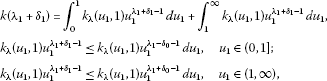and $k\left({\lambda }_{1}-{\delta }_{0}\right)+k\left({\lambda }_{1}+{\delta }_{0}\right)<\mathrm{\infty }$, then by the Lebesgue control convergence theorem (cf. ), it follows $k\left({\lambda }_{1}+{\delta }_{1}\right)=k\left({\lambda }_{1}\right)+o\left(1\right)$ (${\delta }_{1}\to 0$). Assuming that for n (≥2), $k\left({\stackrel{˜}{\lambda }}_{1},\dots ,{\stackrel{˜}{\lambda }}_{n-1}\right)$ is continuous at $\left({\lambda }_{1},\dots ,{\lambda }_{n-1}\right)$, then for $n+1$, by the result of $n=2$, since $k\left({\lambda }_{1}+{\delta }_{1},\dots ,{\lambda }_{n}+{\delta }_{n}\right)$ is finite in a neighborhood of $\left({\lambda }_{1},\dots ,{\lambda }_{n}\right)$, we find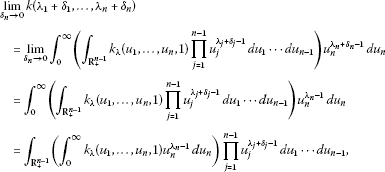then by the assumption for n, it follows

$\underset{{\delta }_{n}\to 0}{lim}k\left({\lambda }_{1}+{\delta }_{1},\dots ,{\lambda }_{n}+{\delta }_{n}\right)=k\left({\lambda }_{1},\dots ,{\lambda }_{n}\right)+o\left(1\right)\phantom{\rule{1em}{0ex}}\left({\delta }_{i}\to 0,i=1,\dots ,n-1\right).$

By mathematical induction, we prove that for $n\in \mathbf{N}\setminus \left\{1\right\}$, $k\left({\stackrel{˜}{\lambda }}_{1},\dots ,{\stackrel{˜}{\lambda }}_{n-1}\right)$ is continuous at $\left({\lambda }_{1},\dots ,{\lambda }_{n-1}\right)$. □

Lemma 4 With the assumptions given in Lemma 2, if there exists $\delta >0$ such that for ${max}_{1\le i\le n-1}\left\{|{\delta }_{i}|\right\}<\delta$, $k\left({\lambda }_{1}+{\delta }_{1},\dots ,{\lambda }_{n-1}+{\delta }_{n-1}\right)\in \mathbf{R}$, ${p}_{i}\in \mathbf{R}\mathrm{\setminus }\left\{0,1\right\}$ ($i=1,\dots ,n$), $0<\epsilon <{min}_{1\le i\le n}\left\{|{p}_{i}|\right\}\delta$, then we have

$\begin{array}{rcl}{I}_{\epsilon }& :=& \epsilon {\int }_{1}^{\mathrm{\infty }}\cdots {\int }_{1}^{\mathrm{\infty }}\left[{\int }_{0}^{1}{x}_{n}^{{\lambda }_{n}+\frac{\epsilon }{{p}_{n}}-1}{k}_{\lambda }\left({x}_{1}{x}_{n},\dots ,{x}_{n-1}{x}_{n},1\right)\phantom{\rule{0.2em}{0ex}}d{x}_{n}\right]\\ ×\prod _{j=1}^{n-1}{x}_{j}^{{\lambda }_{j}-\frac{\epsilon }{{p}_{j}}-1}\phantom{\rule{0.2em}{0ex}}d{x}_{1}\cdots d{x}_{n-1}={k}_{\lambda }+o\left(1\right)\phantom{\rule{1em}{0ex}}\left(\epsilon \to {0}^{+}\right).\end{array}$
(8)

Proof Setting ${x}_{n}^{\mathrm{\prime }}={x}_{n}^{-1}$ in (8), we find

$\begin{array}{rcl}{I}_{\epsilon }& :=& \epsilon {\int }_{1}^{\mathrm{\infty }}\cdots {\int }_{1}^{\mathrm{\infty }}\left[{\int }_{1}^{\mathrm{\infty }}{\left({x}_{n}^{\mathrm{\prime }}\right)}^{-{\lambda }_{n}-\frac{\epsilon }{{p}_{n}}-1}{k}_{\lambda }\left(\frac{{x}_{1}}{{x}_{n}^{\mathrm{\prime }}},\dots ,\frac{{x}_{1}}{{x}_{n}^{\mathrm{\prime }}},1\right)\phantom{\rule{0.2em}{0ex}}d{x}_{n}^{\mathrm{\prime }}\right]\\ ×\prod _{j=1}^{n-1}{x}_{j}^{{\lambda }_{j}-\frac{\epsilon }{{p}_{j}}-1}\phantom{\rule{0.2em}{0ex}}d{x}_{1}\cdots d{x}_{n-1}={k}_{\lambda }+o\left(1\right).\end{array}$

Setting ${u}_{j}={x}_{j}/{x}_{n}^{\mathrm{\prime }}$ ($j=1,\dots ,n-1$) in the above integral, since $\lambda -{\lambda }_{n}={\lambda }_{n}$, we find (replacing ${x}_{n}^{\mathrm{\prime }}$ by ${x}_{n}$)

${I}_{\epsilon }=\epsilon {\int }_{1}^{\mathrm{\infty }}{x}_{n}^{-1-\epsilon }\left[{\int }_{{x}_{n}^{-1}}^{\mathrm{\infty }}\cdots {\int }_{{x}_{n}^{-1}}^{\mathrm{\infty }}{k}_{\lambda }\left({u}_{1},\dots ,{u}_{n-1},1\right)\prod _{j=1}^{n-1}{u}_{j}^{{\lambda }_{j}-\frac{\epsilon }{{p}_{j}}-1}\phantom{\rule{0.2em}{0ex}}d{u}_{1}\cdots d{u}_{n-1}\right]\phantom{\rule{0.2em}{0ex}}d{x}_{n}.$
(9)

Setting ${D}_{j}:=\left\{\left({u}_{1},\dots ,{u}_{n-1}\right)|{u}_{j}\in \left(0,{x}_{n}^{-1}\right),{u}_{k}\in \left(0,\mathrm{\infty }\right)\phantom{\rule{0.25em}{0ex}}\left(k\ne j\right)\right\}$ and

${A}_{j}\left({x}_{n}\right):=\int \cdots {\int }_{{D}_{j}}{k}_{\lambda }\left({u}_{1},\dots ,{u}_{n-1},1\right)\prod _{j=1}^{n-1}{u}_{j}^{{\lambda }_{j}-\frac{\epsilon }{{p}_{j}}-1}\phantom{\rule{0.2em}{0ex}}d{u}_{1}\cdots d{u}_{n-1},$

by (9), it follows

${I}_{\epsilon }\ge {\int }_{{\mathbf{R}}_{+}^{n-1}}{k}_{\lambda }\left({u}_{1},\dots ,{u}_{n-1},1\right)\prod _{j=1}^{n-1}{u}_{j}^{{\lambda }_{j}-\frac{\epsilon }{{p}_{j}}-1}\phantom{\rule{0.2em}{0ex}}d{u}_{1}\cdots d{u}_{n-1}-\epsilon \sum _{j=1}^{n-1}{\int }_{1}^{\mathrm{\infty }}{x}_{n}^{-1}{A}_{j}\left({x}_{n}\right)\phantom{\rule{0.2em}{0ex}}d{x}_{n}.$
(10)

Without loss of generality, we estimate the case of $j=n$, e.t.

${\int }_{1}^{\mathrm{\infty }}{x}_{n}^{-1}{A}_{n-1}\left({x}_{n}\right)\phantom{\rule{0.2em}{0ex}}d{x}_{n}=O\left(1\right)$. In fact, setting $\alpha >0$, such that $|\frac{\epsilon }{{p}_{n-1}}+\alpha |<\delta$, since $-{u}_{n-1}^{\alpha }ln{u}_{n-1}\to 0$ (${u}_{n-1}\to {0}^{+}$), there exists $M>0$ such that $-{u}_{n-1}^{\alpha }ln{u}_{n-1}\le M$ (${u}_{n-1}\in \left(0,1\right]$), and then by the Fubini theorem, it follows

$\begin{array}{rcl}0& \le & {\int }_{1}^{\mathrm{\infty }}{x}_{n}^{-1}{A}_{n-1}\left({x}_{n}\right)\phantom{\rule{0.2em}{0ex}}d{x}_{n}\\ =& {\int }_{1}^{\mathrm{\infty }}{x}_{n}^{-1}\left[{\int }_{{\mathbf{R}}_{+}^{n-2}}{\int }_{0}^{{x}_{n}^{-1}}{k}_{\lambda }\left({u}_{1},\dots ,{u}_{n-1},1\right)\prod _{j=1}^{n-1}{u}_{j}^{{\lambda }_{j}-\frac{\epsilon }{{p}_{j}}-1}\phantom{\rule{0.2em}{0ex}}d{u}_{n-1}\phantom{\rule{0.2em}{0ex}}d{u}_{1}\cdots d{u}_{n-2}\right]\phantom{\rule{0.2em}{0ex}}d{x}_{n}\\ =& {\int }_{0}^{1}{\int }_{{\mathbf{R}}_{+}^{n-2}}{k}_{\lambda }\left({u}_{1},\dots ,{u}_{n-1},1\right)\prod _{j=1}^{n-1}{u}_{j}^{{\lambda }_{j}-\frac{\epsilon }{{p}_{j}}-1}\left({\int }_{1}^{{u}_{n-1}^{-1}}{x}_{n}^{-1}\phantom{\rule{0.2em}{0ex}}d{x}_{n}\right)\phantom{\rule{0.2em}{0ex}}d{u}_{1}\cdots d{u}_{n-1}\\ =& {\int }_{0}^{1}{\int }_{{\mathbf{R}}_{+}^{n-2}}{k}_{\lambda }\left({u}_{1},\dots ,{u}_{n-1},1\right)\prod _{j=1}^{n-1}{u}_{j}^{{\lambda }_{j}-\frac{\epsilon }{{p}_{j}}-1}\left(-ln{u}_{n-1}\right)\phantom{\rule{0.2em}{0ex}}d{u}_{1}\cdots d{u}_{n-1}\\ \le & M{\int }_{0}^{1}{\int }_{{\mathbf{R}}_{+}^{n-2}}{k}_{\lambda }\left({u}_{1},\dots ,{u}_{n-1},1\right)\prod _{j=1}^{n-2}{u}_{j}^{{\lambda }_{j}-\frac{\epsilon }{{p}_{j}}-1}{u}_{n-1}^{{\lambda }_{n-1}-\left(\frac{\epsilon }{{p}_{n-1}}+\alpha \right)-1}\phantom{\rule{0.2em}{0ex}}d{u}_{1}\cdots d{u}_{n-1}\\ \le & M{\int }_{{\mathbf{R}}_{+}^{n-1}}{k}_{\lambda }\left({u}_{1},\dots ,{u}_{n-1},1\right)\prod _{j=1}^{n-2}{u}_{j}^{{\lambda }_{j}-\frac{\epsilon }{{p}_{j}}-1}{u}_{n-1}^{{\lambda }_{n-1}-\left(\frac{\epsilon }{{p}_{n-1}}+\alpha \right)-1}\phantom{\rule{0.2em}{0ex}}d{u}_{1}\cdots d{u}_{n-1}\\ =& M\cdot k\left({\lambda }_{1}-\frac{\epsilon }{{p}_{1}},\dots ,{\lambda }_{n-2}-\frac{\epsilon }{{p}_{n-2}},{\lambda }_{n-1}-\left(\frac{\epsilon }{{p}_{n-1}}+\alpha \right)\right)<\mathrm{\infty }.\end{array}$

Hence by (10), we have

${I}_{\epsilon }\ge {\int }_{{\mathbf{R}}_{+}^{n-1}}{k}_{\lambda }\left({u}_{1},\dots ,{u}_{n-1},1\right)\prod _{j=1}^{n-1}{u}_{j}^{{\lambda }_{j}-\frac{\epsilon }{{p}_{j}}-1}\phantom{\rule{0.2em}{0ex}}d{u}_{1}\cdots d{u}_{n-1}-{o}_{1}\left(1\right).$
(11)

Since by Lemma 3 we find

$\begin{array}{rcl}{I}_{\epsilon }& \le & \epsilon {\int }_{1}^{\mathrm{\infty }}{x}_{n}^{-1-\epsilon }\left[{\int }_{0}^{\mathrm{\infty }}\cdots {\int }_{0}^{\mathrm{\infty }}{k}_{\lambda }\left({u}_{1},\dots ,{u}_{n-1},1\right)\prod _{j=1}^{n-1}{u}_{j}^{{\lambda }_{j}-\frac{\epsilon }{{p}_{j}}-1}\phantom{\rule{0.2em}{0ex}}d{u}_{1}\cdots d{u}_{n-1}\right]\phantom{\rule{0.2em}{0ex}}d{x}_{n}\\ =& {\int }_{0}^{\mathrm{\infty }}\cdots {\int }_{0}^{\mathrm{\infty }}{k}_{\lambda }\left({u}_{1},\dots ,{u}_{n-1},1\right)\prod _{j=1}^{n-1}{u}_{j}^{{\lambda }_{j}-\frac{\epsilon }{{p}_{j}}-1}\phantom{\rule{0.2em}{0ex}}d{u}_{1}\cdots d{u}_{n-1}\\ =& k\left({\lambda }_{1}-\frac{\epsilon }{{p}_{1}},\dots ,{\lambda }_{n-1}-\frac{\epsilon }{{p}_{n-1}}\right)={k}_{\lambda }+{o}_{2}\left(1\right),\end{array}$

then combining with (11), we have (8). □

Lemma 5 Suppose that $n\in \mathbf{N}\mathrm{\setminus }\left\{1\right\}$, ${p}_{i}\in \mathbf{R}\mathrm{\setminus }\left\{0,1\right\}$ ($i=1,\dots ,n$), ${\sum }_{i=1}^{n}\frac{1}{{p}_{i}}=1$, $\frac{1}{{q}_{n}}=1-\frac{1}{{p}_{n}}$, $\left({\lambda }_{1},\dots ,{\lambda }_{n}\right)\in {\mathbf{R}}^{n}$, ${\lambda }_{n}={\sum }_{i=1}^{n-1}{\lambda }_{i}=\frac{\lambda }{2}$, ${k}_{\lambda }\left({x}_{1},\dots ,{x}_{n}\right)$ (≥0) is a measurable function ofλ-degree in ${\mathbf{R}}_{+}^{n}$ such that

${k}_{\lambda }={\int }_{{\mathbf{R}}_{+}^{n-1}}{k}_{\lambda }\left({u}_{1},\dots ,{u}_{n-1},1\right)\prod _{j=1}^{n-1}{u}_{j}^{{\lambda }_{j}-1}\phantom{\rule{0.2em}{0ex}}d{u}_{1}\cdots d{u}_{n-1}\in \mathbf{R}.$

If ${f}_{i}\ge 0$ are measurable functions in ${\mathbf{R}}_{+}$ ($i=1,\dots ,n-1$), ${\stackrel{˜}{k}}_{\lambda }\left({x}_{1},\dots ,{x}_{n}\right):={k}_{\lambda }\left({x}_{1}{x}_{n},\dots ,{x}_{n-1}{x}_{n},1\right)$, then (1) for ${p}_{i}>1$ ($i=1,\dots ,n$), we have

$\begin{array}{rcl}J& :=& {\left\{{\int }_{0}^{\mathrm{\infty }}{x}_{n}^{\frac{\lambda {q}_{n}}{2}-1}{\left[{\int }_{{\mathbf{R}}_{+}^{n-1}}{\stackrel{˜}{k}}_{\lambda }\left({x}_{1},\dots ,{x}_{n}\right)\prod _{i=1}^{n-1}{f}_{i}\left({x}_{i}\right)\phantom{\rule{0.2em}{0ex}}d{x}_{1}\cdots d{x}_{n-1}\right]}^{{q}_{n}}\phantom{\rule{0.2em}{0ex}}d{x}_{n}\right\}}^{\frac{1}{{q}_{n}}}\\ \le & {k}_{\lambda }\prod _{i=1}^{n-1}{\left\{{\int }_{0}^{\mathrm{\infty }}{x}^{{p}_{i}\left(1-{\lambda }_{i}\right)-1}{f}^{{p}_{i}}\left(x\right)\phantom{\rule{0.2em}{0ex}}dx\right\}}^{\frac{1}{{p}_{i}}};\end{array}$
(12)

(2) for $0<{p}_{1}<1$, ${p}_{i}<0$ ($i=2,\dots ,n$), we have the reverse of (12).

Proof (1) For ${p}_{i}>1$ ($i=1,\dots ,n$), by the Hölder inequality (cf. ) and (7), it follows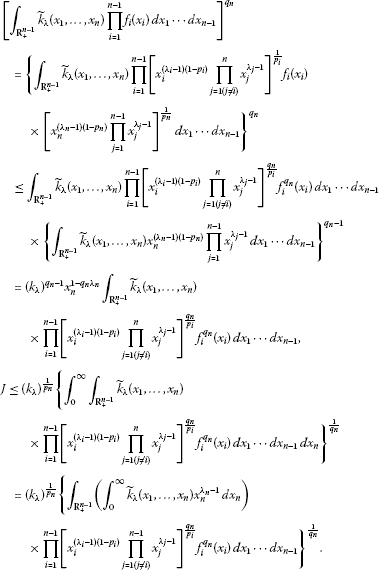(13)

For $n\ge 3$, by the Hölder inequality again, it follows

$\begin{array}{rcl}J& \le & {\left({k}_{\lambda }\right)}^{\frac{1}{{p}_{n}}}\left\{\prod _{i=1}^{n-1}\left[{\int }_{{\mathbf{R}}_{+}^{n-1}}\left({\int }_{0}^{\mathrm{\infty }}{\stackrel{˜}{k}}_{\lambda }\left({x}_{1},\dots ,{x}_{n}\right){x}_{n}^{{\lambda }_{n}-1}\phantom{\rule{0.2em}{0ex}}d{x}_{n}\right)\\ ×{{{x}_{i}^{\left({\lambda }_{i}-1\right)\left(1-{p}_{i}\right)}\prod _{j=1\left(j\ne i\right)}^{n-1}{x}_{j}^{{\lambda }_{j}-1}{f}_{i}^{{p}_{i}}\left({x}_{i}\right)\phantom{\rule{0.2em}{0ex}}d{x}_{1}\cdots d{x}_{n-1}\right]}^{\frac{{q}_{n}}{{p}_{i}}}\right\}}^{\frac{1}{{q}_{n}}}\\ =& {\left({k}_{\lambda }\right)}^{\frac{1}{{p}_{n}}}\prod _{i=1}^{n-1}\left\{{\int }_{0}^{\mathrm{\infty }}\left[{\int }_{{\mathbf{R}}_{+}^{n-1}}{\stackrel{˜}{k}}_{\lambda }\left({x}_{1},\dots ,{x}_{n}\right)\\ ×{{x}_{i}^{{\lambda }_{i}}\prod _{j=1\left(j\ne i\right)}^{n}{x}_{j}^{{\lambda }_{j}-1}\phantom{\rule{0.2em}{0ex}}d{x}_{1}\cdots d{x}_{i-1}\phantom{\rule{0.2em}{0ex}}d{x}_{i+1}\cdots d{x}_{n}\right]{x}_{i}^{{p}_{i}\left(1-{\lambda }_{i}\right)-1}{f}_{i}^{{p}_{i}}\left({x}_{i}\right)\phantom{\rule{0.2em}{0ex}}d{x}_{i}\right\}}^{\frac{1}{{p}_{i}}}\\ =& {\left({k}_{\lambda }\right)}^{\frac{1}{{p}_{n}}}\prod _{i=1}^{n-1}{\left\{{\int }_{0}^{\mathrm{\infty }}{\omega }_{i}\left({x}_{i}\right){x}_{i}^{{p}_{i}\left(1-{\lambda }_{i}\right)-1}{f}_{i}^{{p}_{i}}\left({x}_{i}\right)\phantom{\rule{0.2em}{0ex}}d{x}_{i}\right\}}^{\frac{1}{{p}_{i}}}.\end{array}$
(14)

Then by (7), we have (12). (Note: for $n=2$, we do not use the Hölder inequality again in the above.) (2) For $0<{p}_{1}<1$, ${p}_{i}<0$ ($i=2,\dots ,n$), by the reverse Hölder inequality and in the same way, we obtain the reverses of (12). □

## 3 Main results and applications

With the assumptions given in Lemma 5, setting ${\varphi }_{i}\left(x\right):={x}^{{p}_{{}_{i}\left(1-{\lambda }_{i}\right)-1}}$ ($x\in \left(0,\mathrm{\infty }\right)$; $i=1,\dots ,n$), then we find ${\varphi }_{n}^{1/\left(1-{p}_{n}\right)}\left(x\right)={x}^{{q}_{{}_{n}\lambda _{n}-1}}$. If ${p}_{i}>1$ ($i=1,\dots ,n$), define the following real function spaces: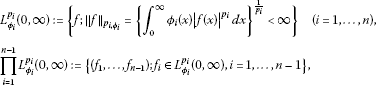and a multiple Hilbert-type integral operator $T:{\prod }_{i=1}^{n-1}{L}_{{\varphi }_{i}}^{{p}_{i}}\left(0,\mathrm{\infty }\right)\to {L}_{{\varphi }_{n}^{1/\left(1-{p}_{n}\right)}}^{{q}_{n}}$ as follows: for $f=\left({f}_{1},\dots ,{f}_{n-1}\right)\in {\prod }_{i=1}^{n-1}{L}_{{\varphi }_{i}}^{{p}_{i}}\left(0,\mathrm{\infty }\right)$,

$\left(Tf\right)\left({x}_{n}\right):={\int }_{{\mathbf{R}}_{+}^{n-1}}{\stackrel{˜}{k}}_{\lambda }\left({x}_{1},\dots ,{x}_{n}\right)\prod _{i=1}^{n-1}{f}_{i}\left({x}_{i}\right)\phantom{\rule{0.2em}{0ex}}d{x}_{1}\cdots d{x}_{n-1},\phantom{\rule{1em}{0ex}}{x}_{n}\in \left(0,\mathrm{\infty }\right).$
(15)

Then by (12), it follows $Tf\in {L}_{{\varphi }_{n}^{1/\left(1-{p}_{n}\right)}}^{{q}_{n}}$, T is bounded, ${\parallel Tf\parallel }_{{q}_{n},{\varphi }_{n}^{1/\left(1-{p}_{n}\right)}}\le {k}_{\lambda }{\prod }_{i=1}^{n-1}{\parallel {f}_{i}\parallel }_{{p}_{i},{\varphi }_{i}}$ and $\parallel T\parallel \le {k}_{\lambda }$, where

$\parallel T\parallel :=\underset{f\in {\prod }_{i=1}^{n-1}{L}_{{\varphi }_{i}}^{{p}_{i}}\left(0,\mathrm{\infty }\right)\left({f}_{i}\ne \theta ,i=1,\dots ,n-1\right)}{sup}\frac{{\parallel Tf\parallel }_{{q}_{n},{\varphi }_{n}^{1/\left(1-{p}_{n}\right)}}}{{\prod }_{i=1}^{n-1}{\parallel {f}_{i}\parallel }_{{p}_{i},{\varphi }_{i}}}.$
(16)

Define the formal inner product of $T\left({f}_{1},\dots ,{f}_{n-1}\right)$ and ${f}_{n}$ as

$\left(T\left({f}_{1},\dots ,{f}_{n-1}\right),{f}_{n}\right):={\int }_{{\mathbf{R}}_{+}^{n}}{\stackrel{˜}{k}}_{\lambda }\left({x}_{1},\dots ,{x}_{n}\right)\prod _{i=1}^{n}{f}_{i}\left({x}_{i}\right)\phantom{\rule{0.2em}{0ex}}d{x}_{1}\cdots d{x}_{n}.$
(17)

Theorem 1 With the assumptions given in Lemma 5, suppose that for any $\left({\lambda }_{1},\dots ,{\lambda }_{n}\right)\in {\mathbf{R}}^{n}$, it satisfies ${\lambda }_{n}={\sum }_{i=1}^{n-1}{\lambda }_{i}=\frac{\lambda }{2}$, and

$0<{k}_{\lambda }={\int }_{{\mathbf{R}}_{+}^{n-1}}{k}_{\lambda }\left({u}_{1},\dots ,{u}_{n-1},1\right)\prod _{j=1}^{n-1}{u}_{j}^{{\lambda }_{j}-1}\phantom{\rule{0.2em}{0ex}}d{u}_{1}\cdots d{u}_{n-1}<\mathrm{\infty }.$
(18)

If ${f}_{i}\left(\ge 0\right)\in {L}_{{\varphi }_{i}}^{{p}_{i}}\left(0,\mathrm{\infty }\right)$, ${\parallel f\parallel }_{{p}_{i,{\varphi }_{i}}}>0$ ($i=1,\dots ,n$), then (i) for ${p}_{i}>1$ ($i=1,\dots ,n$), we have $\parallel T\parallel ={k}_{\lambda }$ and the following equivalent inequalities: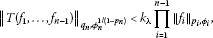(19)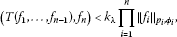(20)

where the constant factor ${k}_{\lambda }$ is the best possible; (ii) for $0<{p}_{1}<1$, ${p}_{i}<0$ ($i=2,\dots ,n$), using the formal symbols in the case of (i), we have the equivalent reverses of (19) and (20) with the same best constant factor.

Proof (i) For all ${p}_{i}>1$, if (12) takes the form of equality, then for $n\ge 3$ in (14), there exist ${C}_{i}$ and ${C}_{k}$ ($i\ne k$) such that they are not all zero ande.t. ${C}_{i}{x}_{i}^{{p}_{i}\left(1-{\lambda }_{i}\right)}{f}_{i}^{{p}_{i}}\left({x}_{i}\right)={C}_{k}{x}_{k}^{{p}_{k}\left(1-{\lambda }_{k}\right)}{f}_{k}^{{p}_{k}}\left({x}_{k}\right)=C$ a.e. in ${\mathbf{R}}_{+}^{n}$. Assuming that ${C}_{i}>0$, then ${x}_{i}^{{p}_{i}\left(1-{\lambda }_{i}\right)-1}{f}_{i}^{{p}_{i}}\left({x}_{i}\right)=C/\left({C}_{i}{x}_{i}\right)$, which contradicts ${\parallel f\parallel }_{{p}_{i,{\varphi }_{i}}}>0$. (Note: for $n=2$, we consider (13) for ${f}_{k}^{{p}_{i}}\left({x}_{k}\right)=1$ in the above.) Hence we have (19). By the Hölder inequality, it follows

$\begin{array}{rcl}\left(Tf,{f}_{n}\right)& =& {\int }_{0}^{\mathrm{\infty }}\left({x}_{n}^{{\lambda }_{n}-\frac{1}{{q}_{n}}}{\int }_{{\mathbf{R}}_{+}^{n-1}}{\stackrel{˜}{k}}_{\lambda }\left({x}_{1},\dots ,{x}_{n}\right)\prod _{i=1}^{n-1}{f}_{i}\left({x}_{i}\right)\phantom{\rule{0.2em}{0ex}}d{x}_{1}\cdots d{x}_{n-1}\right)\left({x}_{n}^{\frac{1}{{q}_{n}}-{\lambda }_{n}}{f}_{n}\left({x}_{n}\right)\right)\phantom{\rule{0.2em}{0ex}}d{x}_{n}\\ \le & {\parallel T\left({f}_{1},\dots ,{f}_{n-1}\right)\parallel }_{{q}_{n},{\varphi }_{n}^{1/\left(1-{p}_{n}\right)}}{\parallel {f}_{n}\parallel }_{{p}_{n},{\varphi }_{n}},\end{array}$
(21)

and then by (19), we have (20). Assuming that (20) is valid, setting

${f}_{n}\left({x}_{n}\right):={x}_{n}^{{q}_{n}{\lambda }_{n}-1}{\left[{\int }_{{\mathbf{R}}_{+}^{n-1}}{\stackrel{˜}{k}}_{\lambda }\left({x}_{1},\dots ,{x}_{n}\right)\prod _{i=1}^{n-1}{f}_{i}\left({x}_{i}\right)\phantom{\rule{0.2em}{0ex}}d{x}_{1}\cdots d{x}_{n-1}\right]}^{{q}_{n}-1},$

then $J={\left\{{\int }_{0}^{\mathrm{\infty }}{x}_{n}^{{p}_{n}\left(1-{\lambda }_{n}\right)-1}{f}_{n}^{{p}_{n}}\left({x}_{n}\right)\phantom{\rule{0.2em}{0ex}}d{x}_{n}\right\}}^{\frac{1}{{q}_{n}}}$. By (12), it follows $J<\mathrm{\infty }$. If $J=0$, then (19) is naturally valid. Assuming that $0, by (20), it follows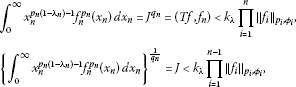and then (19) is valid, which is equivalent to (20).

For $\epsilon >0$ small enough, setting ${\stackrel{˜}{f}}_{i}\left(x\right)$ as: ${\stackrel{˜}{f}}_{i}\left(x\right)=0$, $x\in \left(0,1\right)$; ${\stackrel{˜}{f}}_{i}\left(x\right)={x}^{{\lambda }_{i}-\frac{\epsilon }{{p}_{i}}-1}$, $x\in \left[1,\mathrm{\infty }\right)$ ($i=1,\dots ,n-1$), ${\stackrel{˜}{f}}_{n}\left(x\right)={x}^{{\lambda }_{n}+\frac{\epsilon }{{p}_{n}}-1}$, $x\in \left(0,1\right)$; ${\stackrel{˜}{f}}_{n}\left(x\right)=0$, $x\in \left[1,\mathrm{\infty }\right)$, if there exists $k\le {k}_{\lambda }$ such that (20) is still valid as we replace ${k}_{\lambda }$ by k, then in particular, by Lemma 4, we have

${k}_{\lambda }+o\left(1\right)={I}_{\epsilon }=\epsilon \left(T\left({\stackrel{˜}{f}}_{1},\dots ,{\stackrel{˜}{f}}_{n-1}\right),{\stackrel{˜}{f}}_{n}\right)<\epsilon k\prod _{i=1}^{n}{\parallel {\stackrel{˜}{f}}_{i}\parallel }_{{p}_{i},{\varphi }_{i}}=k$

and ${k}_{\lambda }\le k\left(\epsilon \to {0}^{+}\right)$. Hence $k={k}_{\lambda }$ is the best value of (20). We confirm that the constant factor ${k}_{\lambda }$ in (19) is the best possible, otherwise we can get a contradiction by (21) that the constant factor in (20) is not the best possible. Therefore $\parallel T\parallel ={k}_{\lambda }$.

1. (ii)

For $0<{p}_{1}<1$, ${p}_{i}<0$ ($i=2,\dots ,n$), by using the reverse Hölder inequality and in the same way, we have the equivalent reverses of (19) and (20) with the same best constant factor. □

Example 1 For $\lambda >0$, ${\lambda }_{i}=\frac{\lambda }{{r}_{i}}$ ($i=1,\dots ,n$), ${r}_{n}=2$, ${\sum }_{i=1}^{n}\frac{1}{{r}_{i}}=1$, ${k}_{\lambda }\left({x}_{1},\dots ,{x}_{n}\right)=\frac{1}{{\left({\sum }_{i=1}^{n}{x}_{i}\right)}^{\lambda }}$, by mathematical induction, we can show

${k}_{\lambda }={\int }_{{R}_{+}^{n-1}}\frac{1}{{\left({\sum }_{i=1}^{n-1}{u}_{i}+1\right)}^{\lambda }}\prod _{j=1}^{n-1}{u}_{j}^{\frac{\lambda }{{r}_{j}}-1}\phantom{\rule{0.2em}{0ex}}d{u}_{1}\cdots d{u}_{n-1}=\frac{1}{\mathrm{\Gamma }\left(\lambda \right)}\prod _{i=1}^{n}\mathrm{\Gamma }\left(\frac{\lambda }{{r}_{i}}\right).$
(22)

In fact, for $n=2$, we obtain

${k}_{\lambda }={\int }_{{R}_{+}}\frac{1}{{\left({u}_{1}+1\right)}^{\lambda }}{u}_{1}^{\frac{\lambda }{{r}_{1}}-1}\phantom{\rule{0.2em}{0ex}}d{u}_{1}=\frac{1}{\mathrm{\Gamma }\left(\lambda \right)}\mathrm{\Gamma }\left(\frac{\lambda }{{r}_{1}}\right)\mathrm{\Gamma }\left(\frac{\lambda }{{r}_{2}}\right).$

Assuming that for n (≥2) (22) is valid, then for $n+1$, it follows

$\begin{array}{rcl}{k}_{\lambda }& =& {\int }_{{R}_{+}^{n}}\frac{1}{{\left({\sum }_{i=1}^{n}{u}_{i}+1\right)}^{\lambda }}\prod _{j=1}^{n}{u}_{j}^{\frac{\lambda }{{r}_{j}}-1}\phantom{\rule{0.2em}{0ex}}d{u}_{1}\cdots d{u}_{n}\\ =& {\int }_{{R}_{+}^{n-1}}\prod _{j=2}^{n}{u}_{j}^{\frac{\lambda }{{r}_{j}}-1}\left[{\int }_{{R}_{+}}\frac{1}{{\left[{u}_{1}+\left({\sum }_{i=2}^{n}{u}_{i}+1\right)\right]}^{\lambda }}{u}_{1}^{\frac{\lambda }{{r}_{1}}-1}\phantom{\rule{0.2em}{0ex}}d{u}_{1}\right]\phantom{\rule{0.2em}{0ex}}d{u}_{2}\cdots d{u}_{n}\\ =& {\int }_{{R}_{+}^{n-1}}\frac{1}{{\left({\sum }_{i=2}^{n}{u}_{i}+1\right)}^{\lambda }}\prod _{j=2}^{n}{u}_{j}^{\frac{\lambda }{{r}_{j}}-1}\left[{\int }_{{R}_{+}}\frac{1}{{\left({v}_{1}+1\right)}^{\lambda }}{v}_{1}^{\frac{\lambda }{{r}_{1}}-1}\phantom{\rule{0.2em}{0ex}}d{v}_{1}\right]\phantom{\rule{0.2em}{0ex}}d{u}_{2}\cdots d{u}_{n}\\ =& \frac{\mathrm{\Gamma }\left(\frac{\lambda }{{r}_{1}}\right)\mathrm{\Gamma }\left(\lambda -\frac{\lambda }{{r}_{1}}\right)}{\mathrm{\Gamma }\left(\lambda \right)}{\int }_{{R}_{+}^{n-1}}\frac{1}{{\left({\sum }_{i=2}^{n}{u}_{i}+1\right)}^{\lambda \left(1-\frac{1}{{r}_{1}}\right)}}\prod _{j=2}^{n}{u}_{j}^{\frac{\lambda }{{r}_{j}}-1}\phantom{\rule{0.2em}{0ex}}d{u}_{2}\cdots d{u}_{n}\\ =& \frac{\mathrm{\Gamma }\left(\frac{\lambda }{{r}_{1}}\right)\mathrm{\Gamma }\left(\lambda -\frac{\lambda }{{r}_{1}}\right)}{\mathrm{\Gamma }\left(\lambda \right)}\frac{1}{\mathrm{\Gamma }\left(\lambda -\frac{\lambda }{{r}_{1}}\right)}\prod _{i=2}^{n+1}\mathrm{\Gamma }\left(\frac{\lambda }{{r}_{i}}\right)=\frac{1}{\mathrm{\Gamma }\left(\lambda \right)}\prod _{i=1}^{n+1}\mathrm{\Gamma }\left(\frac{\lambda }{{r}_{i}}\right).\end{array}$

Then by mathematical induction, (22) is valid for $n\in \mathbf{N}\mathrm{\setminus }\left\{1\right\}$.

Example 2 For $\lambda >0$, ${\lambda }_{i}=\frac{\lambda }{{r}_{i}}$ ($i=1,\dots ,n$), ${r}_{n}=2$, ${\sum }_{i=1}^{n}\frac{1}{{r}_{i}}=1$, ${k}_{\lambda }\left({x}_{1},\dots ,{x}_{n}\right)=\frac{1}{{\left({max}_{1\le i\le n}\left\{{x}_{i}\right\}\right)}^{\lambda }}$, by mathematical induction, we can show

${k}_{\lambda }={\int }_{{R}_{+}^{n-1}}\frac{1}{{\left({max}_{1\le i\le n-1}\left\{{u}_{i}\right\}+1\right)}^{\lambda }}\prod _{j=1}^{n-1}{u}_{j}^{\frac{\lambda }{{r}_{j}}-1}\phantom{\rule{0.2em}{0ex}}d{u}_{1}\cdots d{u}_{n-1}=\frac{{\prod }_{i=1}^{n}{r}_{i}}{{\lambda }^{n-1}}.$
(23)

In fact, for $n=2$, we obtain

${k}_{\lambda }={\int }_{{R}_{+}}\frac{{u}_{1}^{\frac{\lambda }{{r}_{1}}-1}}{{\left(max\left\{{u}_{1},1\right\}\right)}^{\lambda }}\phantom{\rule{0.2em}{0ex}}d{u}_{1}={\int }_{0}^{1}{u}_{1}^{\frac{\lambda }{{r}_{1}}-1}\phantom{\rule{0.2em}{0ex}}d{u}_{1}+{\int }_{1}^{\mathrm{\infty }}{u}_{1}^{\frac{-\lambda }{{r}_{2}}-1}\phantom{\rule{0.2em}{0ex}}d{u}_{1}=\frac{1}{\lambda }{r}_{1}{r}_{2}.$

Assuming that for n (≥2), (22) is valid, then for $n+1$, it follows

$\begin{array}{rcl}{k}_{\lambda }& =& {\int }_{{R}_{+}^{n-1}}\prod _{j=2}^{n}{u}_{j}^{\frac{\lambda }{{r}_{j}}-1}\left[{\int }_{0}^{\mathrm{\infty }}\frac{1}{{\left({max}_{1\le i\le n}\left\{{u}_{i},1\right\}\right)}^{\lambda }}{u}_{1}^{\frac{\lambda }{{r}_{1}}-1}\phantom{\rule{0.2em}{0ex}}d{u}_{1}\right]\phantom{\rule{0.2em}{0ex}}d{u}_{2}\cdots d{u}_{n}\\ =& {\int }_{{R}_{+}^{n-1}}\prod _{j=2}^{n}{u}_{j}^{\frac{\lambda }{{r}_{j}}-1}\left[{\int }_{0}^{max\left\{{u}_{2},\dots ,{u}_{n},1\right\}}\frac{1}{{\left({max}_{2\le i\le n}\left\{{u}_{i},1\right\}\right)}^{\lambda }}{u}_{1}^{\frac{\lambda }{{r}_{1}}-1}\phantom{\rule{0.2em}{0ex}}d{u}_{1}\\ +{\int }_{max\left\{{u}_{2},\dots ,{u}_{n},1\right\}}^{\mathrm{\infty }}\frac{1}{{u}_{1}^{\lambda }}{u}_{1}^{\frac{\lambda }{{r}_{1}}-1}\phantom{\rule{0.2em}{0ex}}d{u}_{1}\right]\phantom{\rule{0.2em}{0ex}}d{u}_{2}\cdots d{u}_{n}\\ =& \frac{{r}_{1}^{2}}{\lambda \left({r}_{1}-1\right)}{\int }_{{R}_{+}^{n-1}}\frac{1}{{\left({max}_{2\le i\le n}\left\{{u}_{i},1\right\}\right)}^{\lambda \left(1-\frac{1}{{r}_{1}}\right)}}\prod _{j=2}^{n}{u}_{j}^{\frac{\lambda }{{r}_{j}}-1}\phantom{\rule{0.2em}{0ex}}d{u}_{2}\cdots d{u}_{n}\\ =& \frac{{r}_{1}^{2}}{\lambda \left({r}_{1}-1\right)}{\left(\frac{{r}_{1}}{{r}_{1}-1}\right)}^{n-1}{\int }_{{R}_{+}^{n-1}}\frac{1}{{\left({max}_{2\le i\le n}\left\{{v}_{i},1\right\}\right)}^{\lambda }}\prod _{j=2}^{n}{v}_{j}^{\frac{\lambda }{{r}_{j}}\frac{{r}_{1}}{{r}_{1}-1}-1}\phantom{\rule{0.2em}{0ex}}d{v}_{2}\cdots d{v}_{n}\\ =& \frac{{r}_{1}^{2}}{\lambda \left({r}_{1}-1\right)}{\left(\frac{{r}_{1}}{{r}_{1}-1}\right)}^{n-1}\frac{1}{{\lambda }^{n-1}}\prod _{i=2}^{n+1}\frac{{r}_{1}-1}{{r}_{1}}{r}_{i}=\frac{1}{{\lambda }^{n}}\prod _{i=1}^{n+1}{r}_{i}.\end{array}$

Then by mathematical induction, (23) is valid for $n\in \mathbf{N}\mathrm{\setminus }\left\{1\right\}$.

Example 3 For $\lambda >0$, ${\lambda }_{i}=\frac{-\lambda }{{r}_{i}}$ ($i=1,\dots ,n$), ${r}_{n}=2$, ${\sum }_{i=1}^{n}\frac{1}{{r}_{i}}=1$, ${k}_{\lambda }\left({x}_{1},\dots ,{x}_{n}\right)={\left({min}_{1\le i\le n}\left\{{x}_{i}\right\}\right)}^{\lambda }$, by mathematical induction, we can show

${k}_{-\lambda }={\int }_{{R}_{+}^{n-1}}{\left(min\left\{{u}_{1},\dots ,{u}_{n-1},1\right\}\right)}^{\lambda }\prod _{j=1}^{n-1}{u}_{j}^{\frac{-\lambda }{{r}_{j}}-1}\phantom{\rule{0.2em}{0ex}}d{u}_{1}\cdots d{u}_{n-1}=\frac{{\prod }_{i=1}^{n}{r}_{i}}{{\lambda }^{n-1}}.$
(24)

In fact, for $n=2$, we obtain

${k}_{-\lambda }={\int }_{0}^{1}{u}_{1}^{\frac{\lambda }{{r}_{2}}-1}\phantom{\rule{0.2em}{0ex}}d{u}_{1}+{\int }_{1}^{\mathrm{\infty }}{u}_{1}^{\frac{-\lambda }{{r}_{1}}-1}\phantom{\rule{0.2em}{0ex}}d{u}_{1}=\frac{1}{\lambda }{r}_{1}{r}_{2}.$

Assuming that for n (≥2), (24) is valid, then for $n+1$, it follows

$\begin{array}{rcl}{k}_{-\lambda }& =& {\int }_{{R}_{+}^{n-1}}\prod _{j=2}^{n}{u}_{j}^{\frac{-\lambda }{{r}_{j}}-1}\left[{\int }_{0}^{\mathrm{\infty }}{\left(min\left\{{u}_{1},\dots ,{u}_{n},1\right\}\right)}^{\lambda }{u}_{1}^{\frac{-\lambda }{{r}_{1}}-1}\phantom{\rule{0.2em}{0ex}}d{u}_{1}\right]\phantom{\rule{0.2em}{0ex}}d{u}_{2}\cdots d{u}_{n}\\ =& {\int }_{{R}_{+}^{n-1}}\prod _{j=2}^{n}{u}_{j}^{\frac{-\lambda }{{r}_{j}}-1}\left[{\int }_{0}^{min\left\{{u}_{2},\dots ,{u}_{n},1\right\}}{u}_{1}^{\lambda }{u}_{1}^{\frac{-\lambda }{{r}_{1}}-1}\phantom{\rule{0.2em}{0ex}}d{u}_{1}\\ +{\int }_{min\left\{{u}_{2},\dots ,{u}_{n},1\right\}}^{\mathrm{\infty }}{\left(min\left\{{u}_{2},\dots ,{u}_{n},1\right\}\right)}^{\lambda }{u}_{1}^{\frac{-\lambda }{{r}_{1}}-1}\phantom{\rule{0.2em}{0ex}}d{u}_{1}\right]\phantom{\rule{0.2em}{0ex}}d{u}_{2}\cdots d{u}_{n}\\ =& \frac{{r}_{1}^{2}}{\lambda \left({r}_{1}-1\right)}{\int }_{{R}_{+}^{n-1}}{\left(min\left\{{u}_{2},\dots ,{u}_{n},1\right\}\right)}^{\lambda \left(1-\frac{1}{{r}_{1}}\right)}\prod _{j=2}^{n}{u}_{j}^{\frac{-\lambda \left(1-\frac{1}{{r}_{1}}\right)}{\left(1-\frac{1}{{r}_{1}}\right){r}_{j}}-1}\phantom{\rule{0.2em}{0ex}}d{u}_{2}\cdots d{u}_{n}\\ =& \frac{{r}_{1}^{2}}{\lambda \left({r}_{1}-1\right)}\frac{1}{{\left[\lambda \left(1-\frac{1}{{r}_{1}}\right)\right]}^{n-1}}\prod _{i=2}^{n+1}\left(1-\frac{1}{{r}_{1}}\right){r}_{i}=\frac{1}{{\lambda }^{n}}\prod _{i=1}^{n+1}{r}_{i}.\end{array}$

Then, by mathematical induction, (24) is valid for $n\in \mathbf{N}\mathrm{\setminus }\left\{1\right\}$.

Remarks (i) In particular, for $n=2$ in (20), we have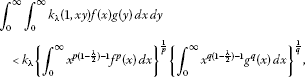(25)

where ${k}_{\lambda }={\int }_{0}^{\mathrm{\infty }}{k}_{\lambda }\left(u,1\right){u}^{\frac{\lambda }{2}-1}\phantom{\rule{0.2em}{0ex}}du>0$ ($\lambda \in \mathbf{R}$) is the best possible. Inequality (25) is an extension of (4) and (8.1.7) in .

1. (ii)

In Examples 1 and 2, by Theorem 1, since for any $\left({\lambda }_{1},\dots ,{\lambda }_{n}\right)\in {\mathbf{R}}^{n}\left({\lambda }_{n}={\sum }_{i=1}^{n}{\lambda }_{i}=\frac{\lambda }{2}\right)$, we obtain $0<{k}_{\lambda }<\mathrm{\infty }$, then we have $\parallel T\parallel ={k}_{\lambda }$ and the equivalent inequalities (19) and (20) with the particular kernels and some equivalent reverses. In Example 3, still using Theorem 1, we find $0<\parallel T\parallel ={k}_{-\lambda }<\mathrm{\infty }$ and the relating particular inequalities.

## References

1. Hardy GH, Littlewood JE, Pólya G: Inequalities. Cambridge University Press, Cambridge; 1934.

2. Mitrinović DS, Pečarić JE, Fink AM: Inequalities Involving Functions and Their Integrals and Derivatives. Kluwer Academic, Boston; 1991.

3. Bicheng Y: A survey of the study of Hilbert-type inequalities with parameters. Adv. Math. 2009, 28(3):257–268.

4. Zhang K: A bilinear inequality. J. Math. Anal. Appl. 2002, 271: 288–296. 10.1016/S0022-247X(02)00104-X

5. Yang B: On a extension of Hilbert’s integral inequality with some parameters. Aust. J. Math. Anal. Appl. 2004., 1(1): Article ID 11

6. Yang B: A new Hilbert’s type integral inequality. Soochow J. Math. 2007, 33(4):849–859.

7. Yang B: A Hilbert-type integral inequality with a non-homogeneous kernel. Journal of Xiamen University (Natural Science) 2009, 48(2):165–169.

8. Yang B: The Norm of Operator and Hilbert-Type Inequalities. Science Press, Beijing; 2009. (China)

9. Hong Y: All-side generalization about Hardy-Hilbert integral inequalities. Acta Math. Sin. 2001, 44(4):619–625.

10. He L, Yu J, Gao M: An extension of Hilbert’s integral inequality. Journal of Shaoguan University (Natural Science) 2002, 23(3):25–30.

11. Yang B: On a multiple Hardy-Hilbert’s integral inequality. Chin. Ann. Math. 2003, 24A(6):25–30.

12. Yang B, Rassias T: On the way of weight coefficient and research for Hilbert-type inequalities. Math. Inequal. Appl. 2003, 6(4):625–658.

13. Yang B, Brnetić I, Krnić M, Pećarić J: Generalization of Hilbert and Hardy-Hilbert integral inequalities. Math. Inequal. Appl. 2005, 8(2):259–272.

14. Bicheng Y: On the norm of an integral operator and applications. J. Math. Anal. Appl. 2006, 321: 182–192. 10.1016/j.jmaa.2005.07.071

15. Bicheng Y: On the norm of a self-adjoint operator and a new bilinear integral inequality. Acta Math. Sin. Engl. Ser. 2007, 23(7):1311–1316. 10.1007/s10114-005-0895-8

16. Benyi A, Choonghong O: Best constant for certain multilinear integral operator. J. Inequal. Appl. 2006., 2006: Article ID 28582

17. Kuang J: Introduction to Real Analysis. Hunan Education Press, Chansha; 1996. (China)

18. Kuang J: Applied Inequalities. Shangdong Science Technic Press, Jinan; 2004. (China)

## Acknowledgements

This work is supported by Guangdong Modern Information Service industry Develop Particularly item 2011 (No. 13090).

## Author information

Authors

### Corresponding author

Correspondence to Qiliang Huang.

### Competing interests

The authors declare that they have no competing interests.

### Authors’ contributions

QH carried out the study, and wrote the manuscript. BY participated in the design of the study, and reformed the manuscript. All authors read and approved the final manuscript.

## Rights and permissions

Reprints and Permissions

Huang, Q., Yang, B. A multiple Hilbert-type integral inequality with a non-homogeneous kernel. J Inequal Appl 2013, 73 (2013). https://doi.org/10.1186/1029-242X-2013-73